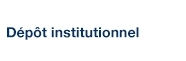# Evaluation of fine root length and diameter measurements obtained using RHIZO image analysis

Bauhus, J. et Messier, C. (1999). « Evaluation of fine root length and diameter measurements obtained using RHIZO image analysis ». Agronomy Journal, 91(1), pp. 142-147.

Fichier(s) associé(s) à ce document :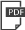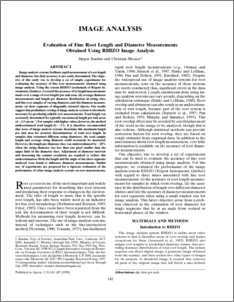Prévisualisation
PDF
Télécharger (233kB)

## Résumé

Image analysis systems facilitate rapid measurement of root length and diameter, but their accuracy is not easily determined. The objective of this study was to develop a set of simple experiments for evaluating the accuracy of fine root measurements obtained using image analysis. Using the system RHIZO (trademark of Regent Instruments, Quebec), we tested the accuracy of (i) length measurements made over a range of root lengths per unit area, (ii) average diameter measurements and length per diameter distributions in string, wire, and fine root samples of varying diameter, and (iii) diameter measurements on short segments of diagonally oriented objects. Our results suggest that preliminary testing of image analysis systems is absolutely necessary for producing reliable root measurements. Total length was accurately determined for typically encountered length per unit areas of <1.5cm cm-2. For samples with higher values, however, the method underestimated total length by >5%. It is therefore recommended that users of image analysis systems determine this maximum length per unit area for accurate determinations of total root length. In samples that contained different string diameters, the total sample length and average string diameter could accurately be measured. However, the length per diameter class was underestimated by >20% when the string diameter was less than one pixel smaller than the upper limit of the diameter class. Adjustment of diameter intervals and increasing the scanner resolution are required to reduce this underestimation. Both the length and the angle of the short segments analyzed were found to influence diameter measurements. Similar sets of experiments are proposed for a rigorous evaluation of the performance of other image analysis systems on root measurements.

Type: Article de revue scientifique fine root length, diameter, measurements, RHIZO image analysis, Faculté des sciences > Département des sciences biologiques Christian Messier 08 janv. 2009 01 nov. 2014 02:08 http://archipel.uqam.ca/id/eprint/1606

## Statistiques

Voir les statistiques sur cinq ans...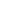RECHERCHER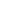PARCOURIR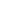LIBRE ACCÈS